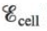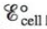# A galvanic cell is based on the following half-reactions at 25°C: Ag + + e − → Ag H 2 O 2 + 2H + + 2e − → 2 H 2 O Predict whether is larger or smaller than for the following cases. a. [ Ag + ] = 1.0 M , [ H 2 O 2 ] = 2.0 M , [ H + ] = 2.0 M b. [ Ag + ] = 2 .0 M , [ H 2 O 2 ] = 1 .0 M , [ H + ] = 1 .0 × 10 − 7 M### Chemistry: An Atoms First Approach

2nd Edition
Steven S. Zumdahl + 1 other
Publisher: Cengage Learning
ISBN: 9781305079243

#### Solutions

Chapter
Section### Chemistry: An Atoms First Approach

2nd Edition
Steven S. Zumdahl + 1 other
Publisher: Cengage Learning
ISBN: 9781305079243
Chapter 17, Problem 67E
Textbook Problem
5 views

## A galvanic cell is based on the following half-reactions at 25°C: Ag + +  e −   →  Ag H 2 O 2 +  2H + +  2e −   →   2 H 2 O Predict whetheris larger or smaller thanfor the following cases.a. [ Ag +   ]   =   1.0   M , [ H 2 O 2 ] =   2.0   M , [ H + ] =   2.0   M b. [ Ag +   ]   =  2 .0   M , [ H 2 O 2 ] =  1 .0   M , [ H + ] =  1 .0 × 10 − 7   M

(a)

Interpretation Introduction

Interpretation:

The chemical half-reactions taking place in a galvanic cell are given. The effect of concentration of reactants and products over Ecell is to be stated.

Concept introduction:

The galvanic cell converts chemical energy into electrical energy while the electrolytic cell converts electrical energy into chemical energy.

The species at anode undergoes oxidation while the species at cathode undergoes reduction and the electrons generated at the anode are transferred through wire to the cathode. The change in concentration of species at anode and cathode also affects the value of Ecell .

To determine: The effect of concentration of reactants and products over the value of Ecell .

### Explanation of Solution

When the value of concentration of reactants is greater than the value of concentration of products, the value of Ecell becomes greater than E°cell (Ecell>E°cell) .

The reaction taking place at cathode is,

H2O2+2H++2e2H2OE°red=+1.78V

The reaction taking place at anode is,

AgAg++eE°ox=0.80V

Multiply oxidation half reaction with the coefficient of 2 and add both the reduction and oxidation half-reaction,

H2O2+2H++2e2H2O2Ag2Ag++2e

The final equation is,

H2O2+2H++2Ag2H2O+2Ag+

The value of overall cell potential is calculated using the formula,

E°cell=E°ox+E°red

Where,

• Eocell is the standard cell potential.

Substitute the values of E°ox and E°red in the above equation

(b)

Interpretation Introduction

Interpretation:

The chemical half-reactions taking place in a galvanic cell are given. The effect of concentration of reactants and products over Ecell is to be stated.

Concept introduction:

The galvanic cell converts chemical energy into electrical energy while the electrolytic cell converts electrical energy into chemical energy.

The species at anode undergoes oxidation while the species at cathode undergoes reduction and the electrons generated at the anode are transferred through wire to the cathode. The change in concentration of species at anode and cathode also affects the value of Ecell .

To determine: The effect of concentration of reactants and products over the value of Ecell .

### Still sussing out bartleby?

Check out a sample textbook solution.

See a sample solution

#### The Solution to Your Study Problems

Bartleby provides explanations to thousands of textbook problems written by our experts, many with advanced degrees!

Get Started

Find more solutions based on key concepts
Which of the following energy systems provides the needed energy for a lifters heave of a heavyweight? the aero...

Nutrition: Concepts and Controversies - Standalone book (MindTap Course List)

What name is given to the nomenclature system for enzymes?

Chemistry for Today: General, Organic, and Biochemistry

Match each substance with its correct description

Human Biology (MindTap Course List)

Why do hot stars look bluer than cool stars?

Horizons: Exploring the Universe (MindTap Course List)

Carry out the arithmetic operations (a) the sum of the measured values 756,37.2,0.83, and 2; (b) the product 0....

Physics for Scientists and Engineers, Technology Update (No access codes included)

What is the difference between a centaur and a NEO?

Foundations of Astronomy (MindTap Course List)